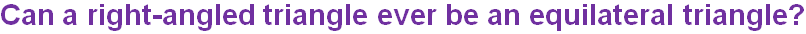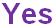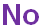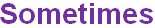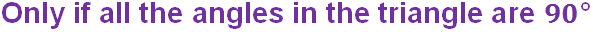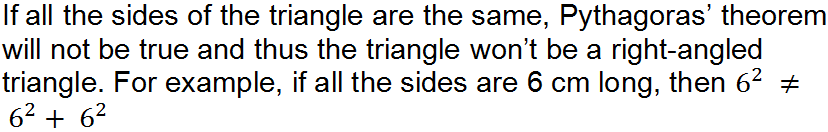Triangular shapes appear in the most unusual places!

# Triangles (F)

Have you ever wondered just how amazing triangles are? With just three sides they are the smallest of the polygon family, but every other polygon can be created from triangles. Three is the smallest number of sides needed to make a rigid, two-dimensional structure. Look at pictures of old bridges such as the Forth Bridge, it is all based on triangles. Test your knowledge of triangle facts in this GCSE Maths quiz!

As its name implies, a triangle has three angles and therefore three sides. The sum of the angles in a triangle is 180o, and so a triangle can have at most, 1 obtuse angle. This is called an obtuse triangle. The boundary between an acute and an obtuse angle is 90o, which is also called a right angle, and so this triangle is a right-angled triangle.

It’s an important one to recognise, as Pythagoras’ Theorem can only be applied to right-angled triangles. If all the angles are acute, then it is an acute triangle.

The only special thing about a scalene triangle is that nothing is equal – no equal sides, no equal angles. Where two sides are equal, there will also be two equal angles. This is an isosceles triangle. This comes from Greek – iso meaning same (think of isobars on weather maps), and sceles meaning legs. If you pronounce the ‘c’ as you say it, it is similar to skeleton. Where does an isosceles triangle form on a skeleton? Stand with your feet apart while you think about it… If you fold an isosceles triangle in half so that the equal sides map onto each other, you will form a right-angled triangle.

The regular triangle is the equilateral, which is based in Latin. Equi is equal, and lateral means sides. Remember the properties of regular polygons – all sides are the same length, and all interior angles are equal. This leads to each angle of an equilateral triangle being 60o.

Question 1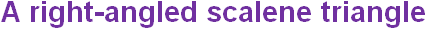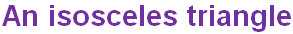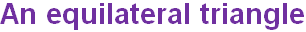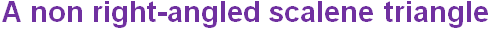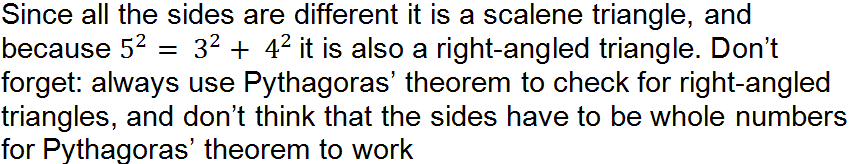Question 2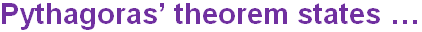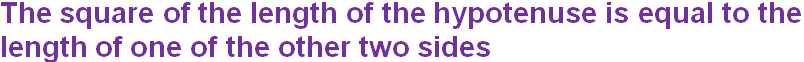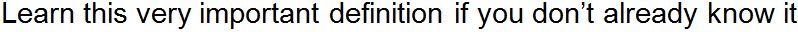Question 3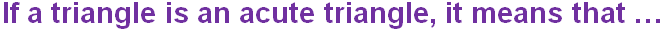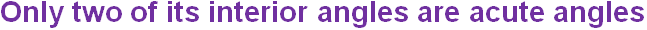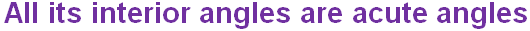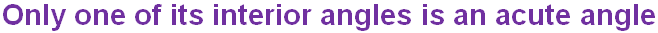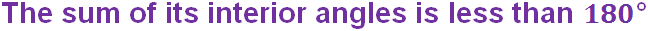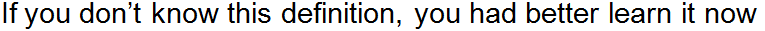Question 4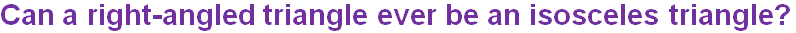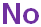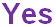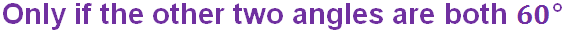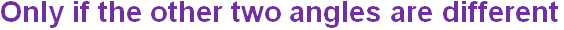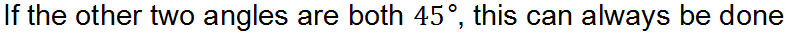Question 5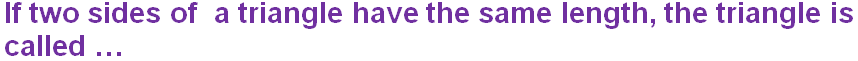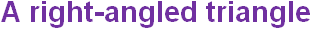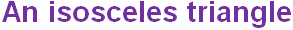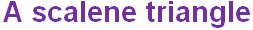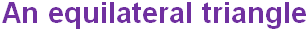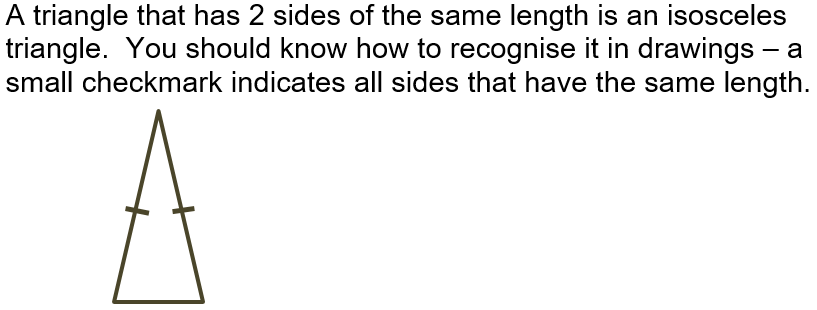Question 6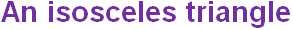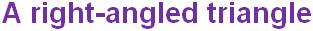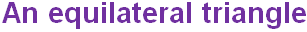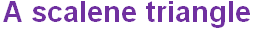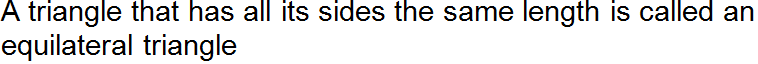Question 7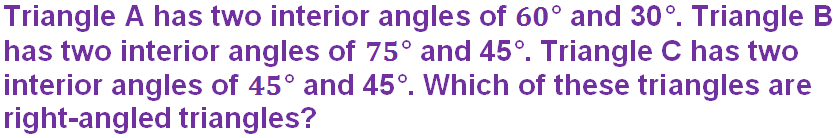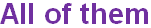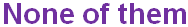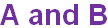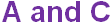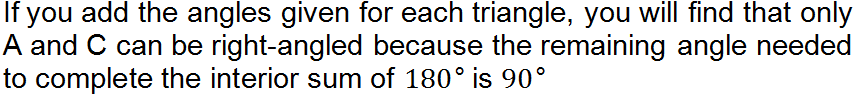Question 8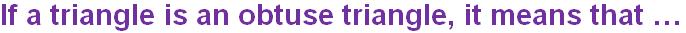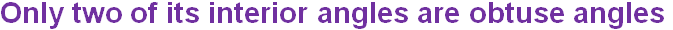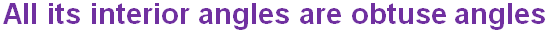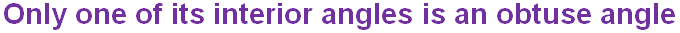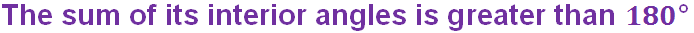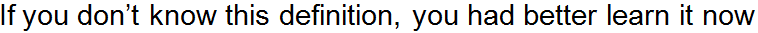Question 9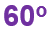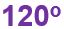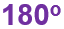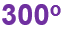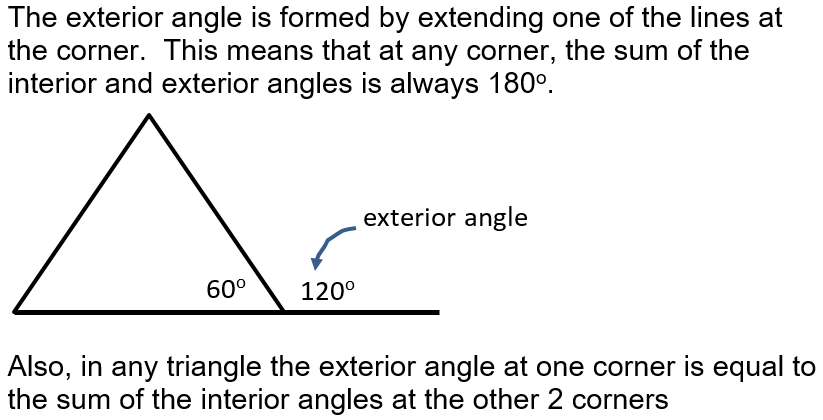Question 10